• +91 9971497814
• info@interviewmaterial.com

# RD Chapter 4- Algebraic Identities Ex-4.3 Interview Questions Answers

### Related Subjects

Question 1 : Find the cube of each of the following binomial expressions: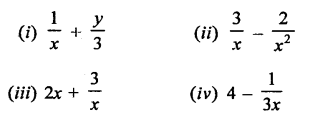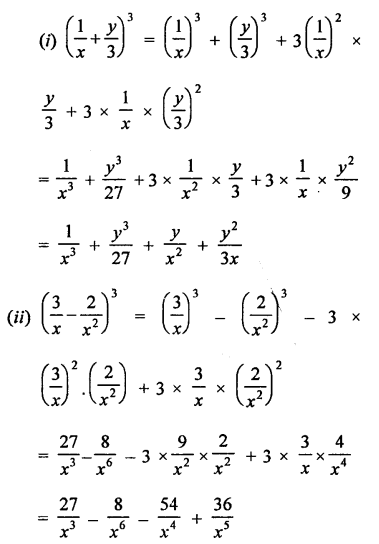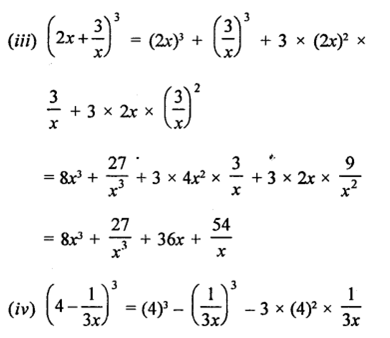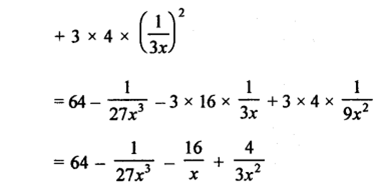Question 2 : If a + b = 10 and ab = 21, find the value of a3 + b3.

a + b = 10, ab = 21
Cubing both sides,
(a + b)3 = (10)3
a3 +63 + 3ab (a + b) = 1000
a3 + b3 + 3 x 21 x 10 = 1000
a3 + b3 + 630 = 1000
a3 + b3 = 1000 – 630 = 370
a3 +b3 = 370

Question 3 : If a – b = 4 and ab = 21, find the value of a3-b3.

a – b = 4, ab= 21
Cubing both sides,
(a –A)3 = (4)3
a3 –b3 – 3ab (a – b) = 64
a3-i3-3×21x4 = 64
a3 – 63 – 252 = 64
a3 – 63 = 64 + 252 =316
a3 –b3 = 316

Question 4 :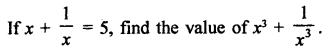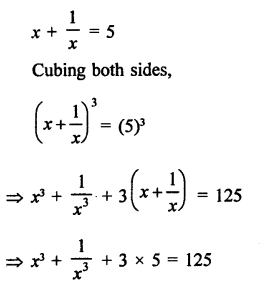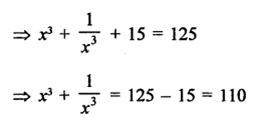Question 5 :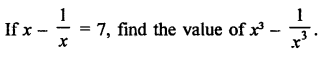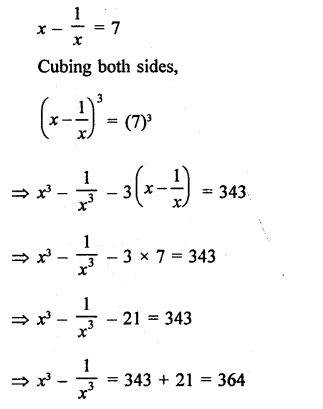Question 6 :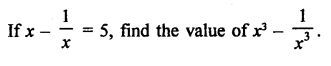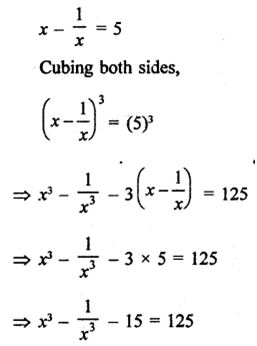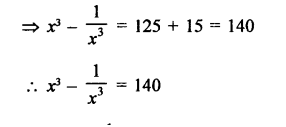Question 7 :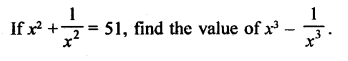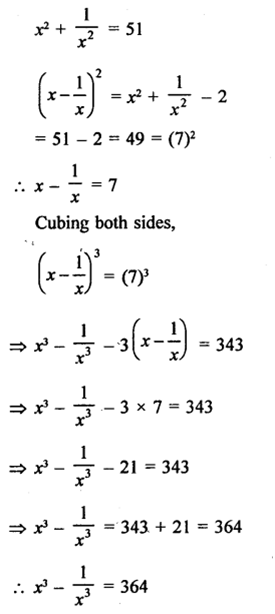Question 8 :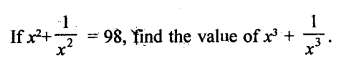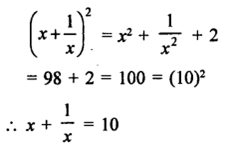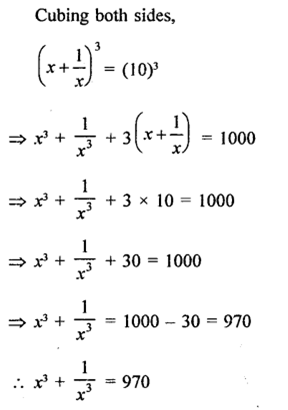Question 9 : If 2x + 3y = 13 and xy = 6, find the value of 8x3 + 21y3.

2x + 3y = 13, xy = 6
Cubing both sides,
(2x + 3y)3 = (13)3
(2x)3 +(3y)3 + 3 x 2x x 3X2x + 3y) = 2197
8x3 +27y3 + 18xy(2x + 3y) = 2197
8x3 +27y3 + 18 x 6 x 13 = 2197
8X3 +27y3 + 1404 = 2197
8x3 + 27y3 = 2197 – 1404 = 793
8x3 +27y3 = 793

Question 10 : If 3x – 2y= 11 and xy = 12, find the value of 27x3 – 8y3.

3x – 2y = 11 and xy = 12 Cubing both sides,
(3x – 2y)3 = (11)3
(3x)3 – (2y)3 – 3 x 3x x 2y(3x – 2y) =1331
27x3 – 8y3 – 18xy(3x -2y) =1331
27x3 – 8y3 – 18 x 12 x 11 = 1331
27x3 – 8y3 – 2376 = 1331
27X3 – 8y3 = 1331 + 2376 = 3707
2x3 –8y3 = 3707

Todays Deals### RD Chapter 4- Algebraic Identities Ex-4.3 Contributorskrishan

Name:
Email:

# Latest News# 9000 interview questions in different categories